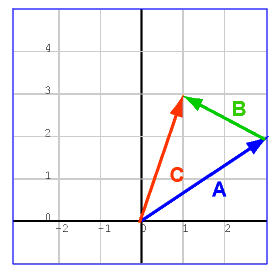# Vector Calculator & Component Resolver

Learning Tool Last Enhanced on February 2, 2013 at 3:19 amThese tools can be used to construct or resolve a vector. All answers are provided to three decimal places. (These tools only support integers and decimals, fractions are not allowed)

*A javascript-enabled browser is required to use this tool.

Vector Addition / Subtraction, given vector components
Be sure to use negative signs for any values that are in the opposite direction.
Magnitude of the vector's horizontal component
Magnitude of the vector's vertical component

Vector Addition / Subtraction, given vectors
Angles should be input in degrees, measured counterclockwise from the horizontal axis / 0 degrees / East.
Magnitude of 1st vector Direction of 1st vector
Magnitude of 2nd vector Direction of 2nd vector
Magnitude of 3rd vector (optional) Direction of 3rd vector

Resolve a Vector into its Components, given magnitude and direction
Convert from polar coordinates to cartesian coordinates
Angles should be input in degrees, measured counterclockwise from the horizontal axis / 0 degrees / East.
Vector Magnitude (R, radius) Vector direction (angle, in degrees)
• Michelle

Calculate the force of gravity on the 1-kg

mass if it were 3.2×106m

above Earth’s surface (that is, if it were one and a half Earth radii from Earth’s center). How do I solve this

• http://www.thecraftycanvas.com/ Anson Han

That’s a Universal Law of Gravity problem. You should be using Fg = G (m1 * m2) / r^2 ; where G = universal gravitation constant, m1 = 1 kg, m2 = mass of earth, r = radius of Earth + 3.2×10^6 m

• Shaiq

can -103i-2j-36k has magnitude of 109 but i want to find direction of magnitude (resultant) vector magnitude. how I can its urgent

• reytchee

What effect do the ff. forces have in a point?
A= 100 N , 30° E of N
B= 200 N , 80° S of E
C= 150 N , 45° S of W
D= 175 N , 25° W of N
E= 50 N, due North

• Techseighter11

1. Two forces A and B are acting on an object. A is 3.0 N directed to north and b is 4.0 N, 30˚ north of east. Find the resultant force acting on the object using the parallelogram method.